## Lattice Multiplication

Lattice multiplication is a predecessor of a more compact long multiplication scheme. It was introduced in Europe in 1202 by Leonardo Fibonacci in his Liber Abaci. The method is so called because it requires a rectangular lattice with one of the diagonals drawn:Each cell of the lattice is split by the diagonal into two parts used to house a 1- or 2-digit number. The number in the cell is the product of two digits, one placed above the cell, the other to its right. For example, this is a one cell lattice that shows the product 8×7: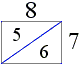A bigger lattice may represent larger numbers. For example, the lattice below is made to help calculate 124576×3857: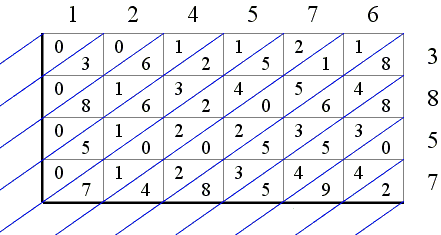The 6 digits of 124576 are placed over six vertical columns, the 4 digits of 3857 generate four rows, and each cell holds the product of the corresponding digits of the two multiplicands. Note that the diagonals split the lattice into (diagonal) bands: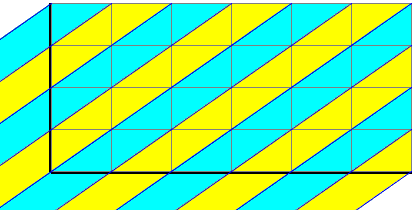The algorithm requires to compute the sums of all the digits in a band and place the result next to the lattice, to the left or below the bottom, as the case may be. The product 124576×3857 leads to the following diagram: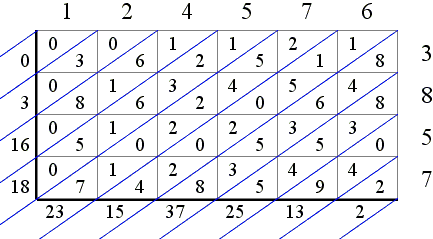The sums are thought of as being ordered around the lattice, first from the top to the bottom and then left to right. Naturally, it may happen that some of these sum are 2-digit numbers, as in the diagram. If all the sums are single digit numbers, the product of the two multiplicands can be read right away by following the sums around the lattice counterclockwise:which tells us that the product 712×23 = 16376.

However, the 2-digit sums cause a complication which is resolved by carrying their first digit to the previous band and adding it to what remains there of the sum placed there previously. So that, for example, for the product 124576×3857, we'll haveIn a simpler case, of say, 4566×257, the lattice is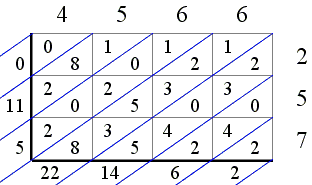and adding the carry yields a one digit number in every cell:so that the product can be read immediately: 4566×257 = 1173462.

In the case of 124576×3857, the third intermediate sum 2 + 8 = 10 takes two digits leading to an additional carry of 1 which is added to the preceding sum 1 + 6 making it 1 + 6 + 1 = 8. This settles the matter giving 124576×3857 = 480489632.

The applet below offers an interactive version of the lattice multiplication. The two multiplicands appear in blue. There digits can be changed by dragging the cursor a little off center of each. The digits can change autonomously so that each will cycle through the values 1, 2, 3, ..., 0, or be made (the default) a part of the integer string so that, for example, if a digit changes from 9 to 0 its neighbor to the left accepts the carry of 1. The length of the multiplicands is controlled by the Initial number of digits spin, but also may change as the number itself changes.

### If you are reading this, your browser is not set to run Java applets. Try IE11 or Safari and declare the site https://www.cut-the-knot.org as trusted in the Java setup.What if applet does not run?• What Is Multiplication?
• Addition and Multiplication Tables in Various Bases
• Peasant Multiplication
• Long Multiplication - an Interactive Gizmo
• Egyptian Multiplication
• Group multiplication of permutations
• Using Math Rules: An Example
• Tables for Multiplication
•